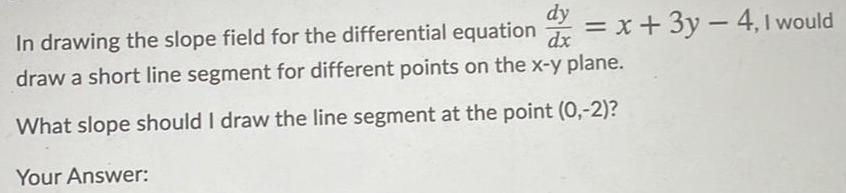Question:

# In drawing the slope field for the differential equation

Last updated: 7/29/2022In drawing the slope field for the differential equation dy/dx= x+3y-4, I would draw a short line segment for different points on the x-y plane. What slope should I draw the line segment at the point (0,-2)? Your Answer: